$\newcommand{\xv}{\mathbf{x}} \newcommand{\Xv}{\mathbf{X}} \newcommand{\yv}{\mathbf{y}} \newcommand{\Yv}{\mathbf{Y}} \newcommand{\zv}{\mathbf{z}} \newcommand{\av}{\mathbf{a}} \newcommand{\Wv}{\mathbf{W}} \newcommand{\wv}{\mathbf{w}} \newcommand{\betav}{\mathbf{\beta}} \newcommand{\gv}{\mathbf{g}} \newcommand{\Hv}{\mathbf{H}} \newcommand{\dv}{\mathbf{d}} \newcommand{\Vv}{\mathbf{V}} \newcommand{\vv}{\mathbf{v}} \newcommand{\Uv}{\mathbf{U}} \newcommand{\uv}{\mathbf{u}} \newcommand{\tv}{\mathbf{t}} \newcommand{\Tv}{\mathbf{T}} \newcommand{\Sv}{\mathbf{S}} \newcommand{\Gv}{\mathbf{G}} \newcommand{\zv}{\mathbf{z}} \newcommand{\Zv}{\mathbf{Z}} \newcommand{\Norm}{\mathcal{N}} \newcommand{\muv}{\boldsymbol{\mu}} \newcommand{\sigmav}{\boldsymbol{\sigma}} \newcommand{\phiv}{\boldsymbol{\phi}} \newcommand{\Phiv}{\boldsymbol{\Phi}} \newcommand{\Sigmav}{\boldsymbol{\Sigma}} \newcommand{\Lambdav}{\boldsymbol{\Lambda}} \newcommand{\half}{\frac{1}{2}} \newcommand{\argmax}{\underset{#1}{\operatorname{argmax}}} \newcommand{\argmin}{\underset{#1}{\operatorname{argmin}}} \newcommand{\dimensionbar}{\underset{#1}{\operatorname{|}}} \newcommand{\grad}{\mathbf{\nabla}} \newcommand{\ebx}{e^{\betav_{#1}^T \xv_n}} \newcommand{\eby}{e^{y_{n,#1}}} \newcommand{\Tiv}{\mathbf{Ti}} \newcommand{\Fv}{\mathbf{F}} \newcommand{\ones}{\mathbf{1}_{#1}}$

# Linear Dimensionality Reduction¶

## Principal Components Analysis (PCA)¶

Principal Components Analysis (PCA) is a way to find and use directions in the data space along which data samples vary the most.

Assume samples have $D$ attributes, meaning each sample is $D$-dimensional. We want to project each sample to a smaller space, of dimension $M$ such that the variance of the projected data has maximum variance.

Let's assume each sample $\xv_n$ has zero mean. For $M=1$, we want the direction vector (unit length) $\uv_1$ that maximizes the variance of each projected sample. This variance is $$\frac{1}{N} \sum_{n=1}^N (\uv_1^T \xv_n)^2 = \uv_1^T \Sv \uv_1$$ where $$\Sv = \frac{1}{N} \sum_{n=1}^N \xv_n \xv_n^T$$ To maximize $\uv_1^T \Sv \uv_1$ in a non-trivial way, we constrain $\uv_1^T \uv_1 = 1$. This constraint is added with a Lagrange multipler so that we want $\uv_1$ that maximizes $$\uv_1^T \Sv \uv_1+ \lambda_1(1-\uv_1^T \uv_1)$$ Setting the derivative of this with respect to $\uv_1$ to zero we find that $$\Sv \uv_1 = \lambda_1 \uv_1$$ so $\uv_1$ is an eigenvector of $\Sv$ and $\lambda_1$ is an eigenvalue that is the variance of the projected samples.

Additional directions, all orthogonal to each other, are found by the eigendecomposition of $\Sv$, or, equivalently, the singular value decomposition of data sample matrix $\Xv$ with mean zero.
$$\Uv \Sigmav \Vv^T = \Xv$$ The columns of $\Vv$ are the eigenvectors of $\Sv$ and the elements of the diagonal matrix $\Sigmav$ are the square root of the eigenvalues.

X = X - np.mean(X,axis=0)
U,s,V = np.linalg.svd(X)
V = V.T



Then, to project onto the eigenvectors, just

proj = X @ V

Let's generate some two-dimensional samples from a Normal distribution with mean [0,4] and covariance matrix $\Sigma=\begin{bmatrix} 0.9 & 0.8\\ 0.8 & 0.9 \end{bmatrix}$. Then we will calculate the svd of the samples and project the samples to the two eigenvectors.

In :
import numpy as np
import matplotlib.pyplot as plt
%matplotlib inline

In :
def mvNormalRand(n,mean,sigma):
mean = np.array(mean) # to allow entry of values as simple lists
sigma = np.array(sigma)
X = np.random.normal(0,1,n*len(mean)).reshape((n,len(mean)))
return np.dot(X, np.linalg.cholesky(sigma)) + mean

N = 200
data = mvNormalRand(N,[0,4],[[0.9,0.8],[0.8,0.9]])
data.shape

Out:
(200, 2)
In :
means = np.mean(data, axis=0)
datan = data - means

U,S,V = np.linalg.svd(datan)
V = V.T
V.shape

Out:
(2, 2)
In :
V

Out:
array([[-0.98034865,  0.19727273],
[-0.19727273, -0.98034865]])
In :
def drawline(v, means, length, color, label):
p1 = means - v * length / 2
p2 = means + v * length / 2
plt.plot([p1, p2], [p1, p2], label=label, color=color, linewidth=2)

def plotOriginalAndTransformed(data, V):
plt.figure(figsize=(10, 5))
plt.subplot(1, 2, 1)
plt.plot(data[:, 0], data[: ,1], '.')
means = np.mean(data, axis=0)
drawline(V[:, 0], means, 8, "red", "First")
drawline(V[:, 1], means, 8, "green", "Second")
leg = plt.legend()
plt.axis('equal')
plt.gca().set_aspect('equal')

plt.subplot(1, 2, 2)
proj = (data - means) @ V
plt.plot(proj[:, 0], proj[:, 1], '.')
plt.axis('equal')
plt.gca().set_aspect('equal')
plt.xlabel("First")
plt.ylabel("Second")
plt.title("Projected to First and Second Singular Vectors");

plotOriginalAndTransformed(data,V)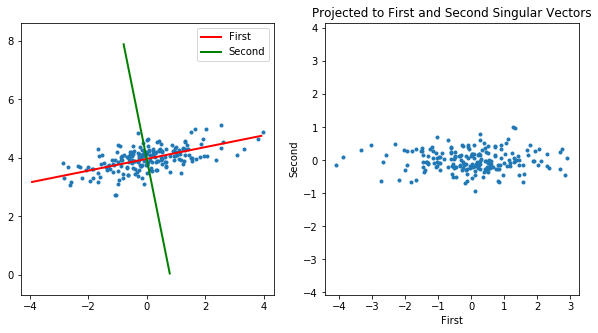Now, if we have two classes of data, we might be able to classify the data well with just the projection onto just one eigenvector. Could be either eigenvector.

First, with second class having mean [-5,3] and $\Sigma=\begin{bmatrix} 0.9 & 0.8\\ -0.8 & 0.9 \end{bmatrix}$.

In :
N = 200
data1 = mvNormalRand(N, [0, 4], [[0.9 ,0.8], [0.8, 0.9]])
data2 = mvNormalRand(N, [-5, 3], [[0.9, 0.8], [-0.8, 0.9]])
data = np.vstack((data1, data2))

means = np.mean(data, axis=0)

U, S ,V = np.linalg.svd(data  -means)
V = V.T

plotOriginalAndTransformed(data, V)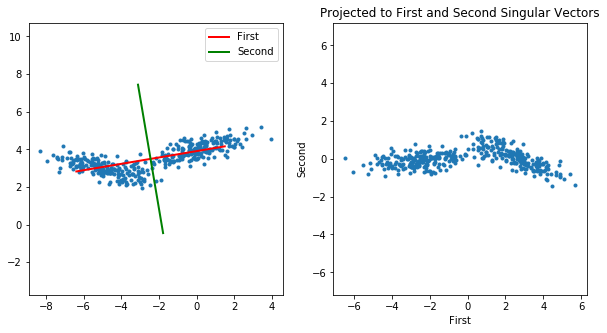And again, with first class $\Sigma=\begin{bmatrix} 0.9 & 0.2\\ 0.2 & 20 \end{bmatrix}$ and second class having $\Sigma=\begin{bmatrix} 0.9 & 0.2\\ -0.2 & 20 \end{bmatrix}$.

In :
N = 200
data1 = mvNormalRand(N, [0, 4], [[0.9 ,0.2], [0.2, 0.9]])
data2 = mvNormalRand(N, [-5, 3], [[0.9, 0.2], [-0.2, 20]])
data = np.vstack((data1, data2))

means = np.mean(data, axis=0)

U, S, V = np.linalg.svd(data - means)
V = V.T

plotOriginalAndTransformed(data, V)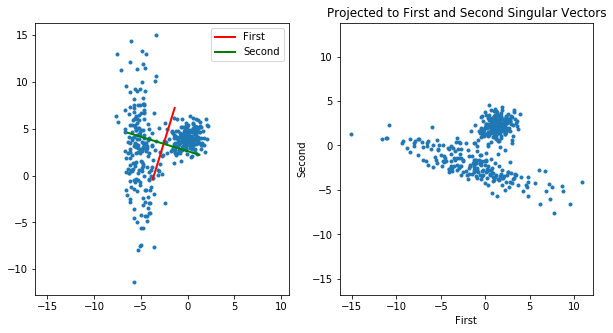## Sammon Mapping¶

Introductions to Sammon Mapping are found at

A Sammon Mapping is one that maps each data sample $d_i$ to a location in two dimensions, $p_i$, such that distances between pairs of points are preserved. The objective defined by Sammon is to minimize the squared difference in distances between pairs of data points and their projections through the use of an objective function like $$\sum_{i=1}^{N-1} \sum_{j=i+1}^N \left (\frac{||d_i - d_j||}{s} - ||p_i - p_j|| \right )^2$$ The typical Sammon Mapping algorithm does a gradient descent on this function by adjusting all of the two-dimensional points $p_{ij}$. Each iteration requires computing all pairwise distances.

One way to decrease this amount of work is to just work with a subset of points, perhaps picked randomly. To display all points, we just find an explicit mapping (function) that projects a data sample to a two-dimensional point. Let's call this $f$, so $f(d_i) = p_i$. For now, let's just use a linear function for $f$, so $$f(d_i) = d_i^T \theta$$ where $\theta$ is a $D\times 2$ matrix of coefficients.

To do this in python, let's start with calculating all pairwise distances. Let $X$ be our $N\times D$ matrix of data samples, one per row. We can use a list comprehension to calculate the distance between each row in $X$ and each of the rows following that row.

In :
X = np.array([[0, 1], [4, 5], [10, 20]])
X

Out:
array([[ 0,  1],
[ 4,  5],
[10, 20]])
In :
N = X.shape # number of rows
[(i, j) for i in range(N - 1) for j in range(i + 1, N)]

Out:
[(0, 1), (0, 2), (1, 2)]
In :
[X[i, :] - X[j, :] for i in range(N - 1) for j in range(i + 1, N)]

Out:
[array([-4, -4]), array([-10, -19]), array([ -6, -15])]
In :
np.array([X[i, :] - X[j, :] for i in range(N - 1) for j in range(i + 1, N)])

Out:
array([[ -4,  -4],
[-10, -19],
[ -6, -15]])

To convert these differences to distances, just

In :
diffs = np.array([X[i, :] - X[j, :] for i in range(N - 1) for j in range(i + 1 ,N)])
np.sqrt(np.sum(diffs * diffs, axis=1))

Out:
array([ 5.65685425, 21.47091055, 16.15549442])

And to calculate the projection, a call to np.dot is all that is needed. Let's make a function to do the projection, and one to convert differences to distances.

In :
def diffToDist(dX):
return np.sqrt(np.sum(dX * dX, axis=1))

def proj(X, theta):
return X @ theta

In :
diffToDist(diffs)

Out:
array([ 5.65685425, 21.47091055, 16.15549442])
In :
proj(X, np.array([[1 ,0.2], [0.3, 0.8]]))

Out:
array([[ 0.3,  0.8],
[ 5.5,  4.8],
[16. , 18. ]])

Now, to follow the negative gradient of the objective function, we need its gradient, with respect to $\theta$. With a little work, you can derive it to find \begin{align*} \nabla_\theta &= \frac{1}{2} \sum_{i=1}^{N-1} \sum_{j=i+1}^N \left (\frac{||d_i - d_j||}{s} - ||p_i - p_j|| \right )^2 \\ &= 2 \frac{1}{2} \sum_{i=1}^{N-1} \sum_{j=i+1}^N \left (\frac{||d_i - d_j||}{s} - ||f(d_i;\theta) - f(d_j;\theta)|| \right ) (-1) \nabla_\theta ||f(d_i;\theta) - f(d_j;\theta)||\\ &= - \sum_{i=1}^{N-1} \sum_{j=i+1}^N \left (\frac{||d_i - d_j||}{s} - ||f(d_i;\theta) - f(d_j;\theta)|| \right ) \frac{(d_i-d_j)^T (p_i - p_j)}{||p_i - p_j||} \end{align*}

So, we need to keep the differences around, in addition to the distances. First, let's write a function for the objective function, so we can monitor it, to make sure we are decrease it with each iteration. Let's multiply by $1/N$ so the values we get don't grow huge with large $N$.

In :
def objective(X, proj, theta, s):
N = X.shape
P = proj(X, theta)
dX = np.array([X[i, :] - X[j, :] for i in range(N - 1) for j in range(i + 1, N)])
dP = np.array([P[i, :] - P[j, :] for i in range(N - 1) for j in range(i + 1, N)])
return 1 / N * np.sum( (diffToDist(dX) / s - diffToDist(dP))**2)


Now for the gradient \begin{align*} \nabla_\theta &= - \sum_{i=1}^{N-1} \sum_{j=i+1}^N \left (\frac{||d_i - d_j||}{s} - ||f(d_i;\theta) - f(d_j;\theta)|| \right ) \frac{(d_i-d_j)^T (p_i - p_j)}{||p_i - p_j||} \end{align*}

In :
def gradient(X, proj, theta, s):
N = X.shape
P = proj(X, theta)
dX = np.array([X[i, :] - X[j, :] for i in range(N - 1) for j in range(i + 1, N)])
dP = np.array([P[i, :] - P[j, :] for i in range(N - 1) for j in range(i + 1, N)])
distX = diffToDist(dX)
distP = diffToDist(dP)
return -1 / N * (((distX/s - distP) / distP).reshape((-1,1)) * dX).T @ dP


This last line has the potential for dividing by zero! Let's avoid this, in a very ad-hoc manner, by replacing zeros in distP by its smallest nonzero value

In :
def gradient(X, proj, theta, s):
N = X.shape
P = proj(X, theta)
dX = np.array([X[i, :] - X[j, :] for i in range(N - 1) for j in range(i + 1, N)])
dP = np.array([P[i, :] - P[j, :] for i in range(N - 1) for j in range(i + 1, N)])
distX = diffToDist(dX)
distP = diffToDist(dP)
minimumNonzero = np.min(distP[distP > 0])
distP[distP == 0] = minimumNonzero
return -1 / N * (((distX/s - distP) / distP).reshape((-1,1)) * dX).T @ dP

In :
n = 8
X = np.random.multivariate_normal([2, 3], 0.5 * np.eye(2), n)
X = np.vstack((X, np.random.multivariate_normal([1, -1], 0.2  *np.eye(2), n)))
X = X - np.mean(X, axis=0)
s = 0.5 * np.sqrt(np.max(np.var(X, axis=0)))
print('s', s)

# theta = np.random.uniform(-1,1,(2,2))
# theta = np.eye(2) + np.random.uniform(-0.1,0.1,(2,2))
u, svalues, v = np.linalg.svd(X)
v = v.T
theta = v[:, :2]

nIterations = 10
vals = []
for i in range(nIterations):
theta -= 0.001 * gradient(X, proj, theta, s)
v = objective(X, proj, theta, s)
vals.append(v)

# print('X\n',X)
# print('P\n',proj(X,theta))
print('theta\n', theta)
plt.figure(figsize=(10, 15))
plt.subplot(3, 1, (1, 2))
P = proj(X, theta)
mn = 1.1 * np.min(X)
mx = 1.1 * np.max(X)
plt.axis([mn, mx, mn, mx])
#strings = [chr(ord('a')+i) for i in range(X.shape)]
strings = [i for i in range(X.shape)]
for i in range(X.shape):
plt.text(X[i, 0], X[i, 1], strings[i], color='black', size=15)
for i in range(P.shape):
plt.text(P[i, 0], P[i, 1], strings[i], color='red', size=15)
plt.title('2D data, Originals in black')

plt.subplot(3, 1, 3)
plt.plot(vals)
plt.ylabel('Objective Function');

s 0.9700388238644607
theta
[[-0.31540962  0.95200363]
[-0.96526407 -0.31104457]]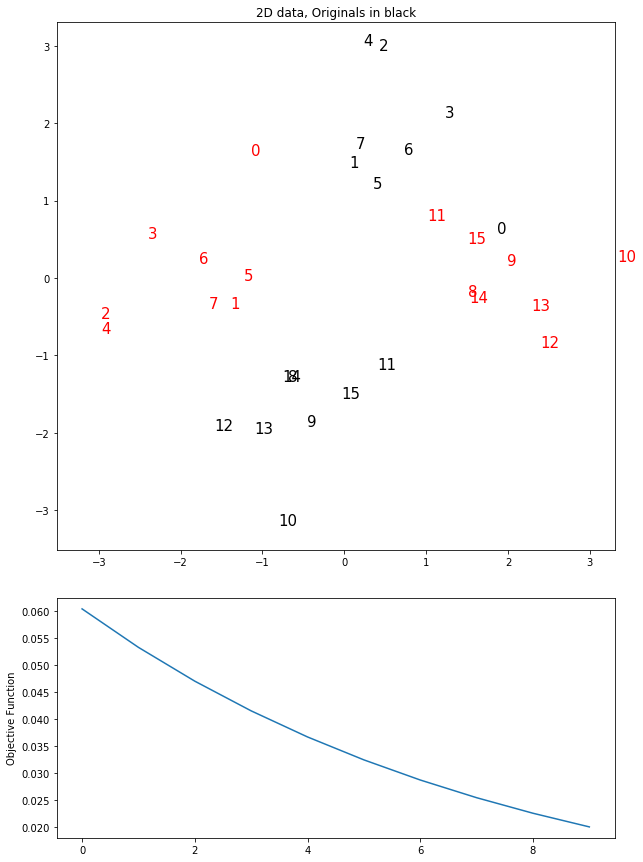Let's watch the mapping develop. One way to do this is to save the values of $\theta$ after each iteration, then use interact to step through the interations.

In :
from ipywidgets import interact

@interact(x=10)
def f(x):
return x, x**2

(10, 100)
In :
n = 10
X = np.random.multivariate_normal([2, 3], 0.5 * np.eye(2), n)
X = np.vstack((X, np.random.multivariate_normal([1, -1], 0.2 * np.eye(2), n)))
X = X - np.mean(X, axis=0)
s = 0.5 * np.sqrt(np.max(np.var(X, axis=0)))
print('s', s)

u, svalues, v = np.linalg.svd(X)
V = v.T
theta = V[:, :2]

theta = (np.random.uniform(size=((2, 2))) - 0.5) * 10

thetas = [theta] # store all theta values

nIterations = 200
vals = []
for i in range(nIterations):
theta = theta - 0.02 * gradient(X, proj, theta, s)
v = objective(X, proj, theta, s)
thetas.append(theta.copy())
vals.append(v)

mn = 1.5 * np.min(X)
mx = 1.5 * np.max(X)

strings = [i for i in range(X.shape)]

@interact(iteration=(0, nIterations-1, 1))
def plotIteration(iteration):
#plt.cla()
plt.figure(figsize=(12, 12));
theta = thetas[iteration]
val = vals[iteration]
P = proj(X, theta)
plt.axis([mn, mx, mn, mx])
for i in range(X.shape):
plt.text(X[i, 0], X[i, 1], strings[i], color='black', size=15)
for i in range(P.shape):
plt.text(P[i, 0], P[i, 1], strings[i], color='red', size=15)
plt.title('2D data, Originals in black. Objective={:.3f} Iter={}'.format(val, iteration))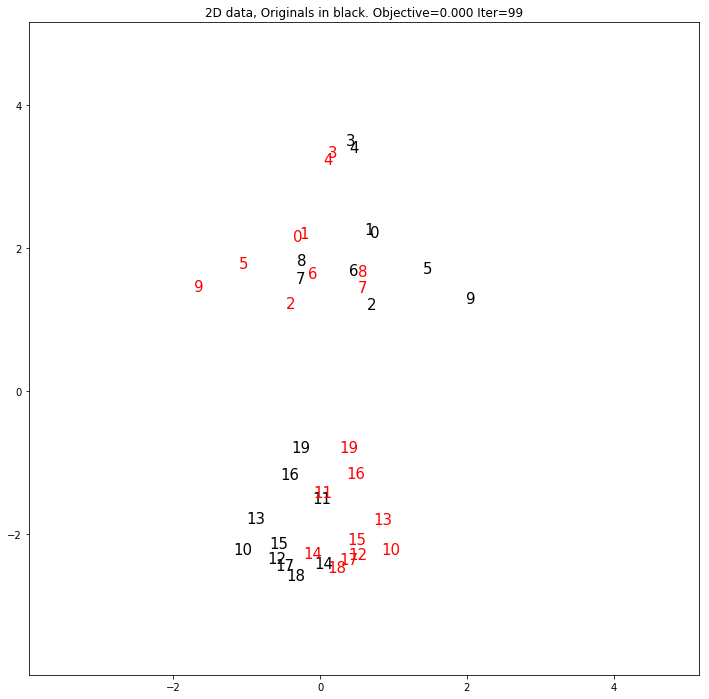# Examples of Linear Dimensionality Reduction¶

## Principal Components Analysis (PCA)¶

Let's apply PCA to the MNIST digits.

In :
import numpy as np
import matplotlib.pyplot as plt
%matplotlib inline
import gzip
import pickle

In :
with gzip.open('mnist.pkl.gz', 'rb') as f:
train_set, valid_set, test_set = pickle.load(f, encoding='latin1')

Xtrain = train_set
Ttrain = train_set.reshape((-1,1))

Xtest = test_set
Ttest = test_set.reshape((-1,1))

Xtrain.shape, Ttrain.shape, Xtest.shape, Ttest.shape

Out:
((50000, 784), (50000, 1), (10000, 784), (10000, 1))
In :
mean = Xtrain.mean(axis=0)

In :
plt.plot(mean)

Out:
[<matplotlib.lines.Line2D at 0x7f5f82712cc0>]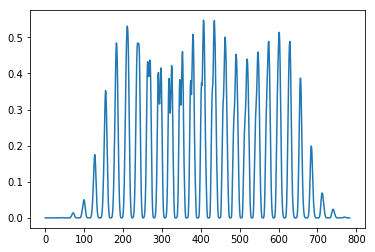In :
plt.imshow(mean.reshape((28,28)), cmap='gray', interpolation='nearest')

Out:
<matplotlib.image.AxesImage at 0x7f5f82673d68>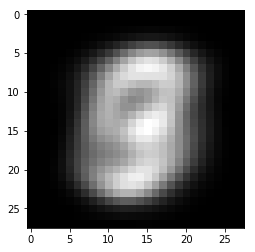In :
mean.shape

Out:
(784,)
In :
U, S, V = np.linalg.svd(Xtrain - mean, full_matrices=False)
V = V.T

In :
U.shape, S.shape, V.shape

Out:
((50000, 784), (784,), (784, 784))
In :
plt.plot(S);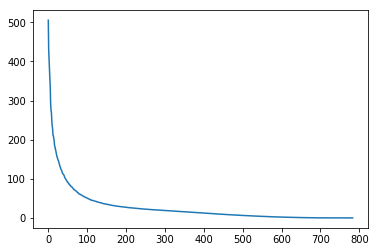In :
plt.imshow(V[:,1].reshape((28,28)), cmap='gray')
plt.colorbar()

Out:
<matplotlib.colorbar.Colorbar at 0x7f5f82751da0>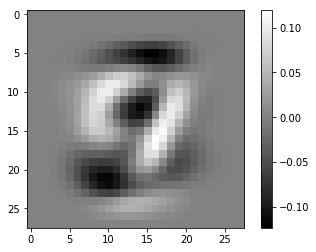In :
X = Xtrain[:40,:]
X = X - mean
X.shape

Out:
(40, 784)
In :
p = X @ V[:, 1:2]
np.hstack((Ttrain[:40,:].astype(int), p))

Out:
array([[ 5.00000000e+00, -1.24686456e+00],
[ 0.00000000e+00, -1.25165141e+00],
[ 4.00000000e+00,  1.54788339e+00],
[ 1.00000000e+00, -2.29611897e+00],
[ 9.00000000e+00,  2.87207937e+00],
[ 2.00000000e+00,  8.54624748e-01],
[ 1.00000000e+00, -5.71480453e-01],
[ 3.00000000e+00, -1.24150157e+00],
[ 1.00000000e+00, -2.79385388e-01],
[ 4.00000000e+00,  1.85472143e+00],
[ 3.00000000e+00, -2.52480197e+00],
[ 5.00000000e+00, -9.01752472e-01],
[ 3.00000000e+00, -3.26690817e+00],
[ 6.00000000e+00,  6.00088120e-01],
[ 1.00000000e+00, -8.56807828e-01],
[ 7.00000000e+00,  3.42006087e+00],
[ 2.00000000e+00,  2.84147263e-03],
[ 8.00000000e+00, -9.59410429e-01],
[ 6.00000000e+00, -7.64632046e-01],
[ 9.00000000e+00,  3.56650263e-01],
[ 4.00000000e+00,  1.97236419e+00],
[ 0.00000000e+00, -1.49304175e+00],
[ 9.00000000e+00,  8.39059532e-01],
[ 1.00000000e+00, -1.98540640e+00],
[ 1.00000000e+00, -1.11565435e+00],
[ 2.00000000e+00, -4.15563583e+00],
[ 4.00000000e+00,  1.69763064e+00],
[ 3.00000000e+00, -2.45021486e+00],
[ 2.00000000e+00,  8.05178881e-01],
[ 7.00000000e+00,  5.33476591e-01],
[ 3.00000000e+00, -1.79209903e-01],
[ 8.00000000e+00, -2.47361088e+00],
[ 6.00000000e+00,  3.46762240e-01],
[ 9.00000000e+00,  7.73301005e-01],
[ 0.00000000e+00, -1.01077473e+00],
[ 5.00000000e+00, -7.43270159e-01],
[ 6.00000000e+00, -9.05223787e-01],
[ 0.00000000e+00, -1.03844571e+00],
[ 7.00000000e+00,  2.28403592e+00],
[ 6.00000000e+00,  1.12173533e+00]])
In :
Ttrain[:20]

Out:
array([,
,
,
,
,
,
,
,
,
,
,
,
,
,
,
,
,
,
,
])
In :
V[:, 2:3].T @ V[:, 1:2]

Out:
array([[0.]], dtype=float32)
In :
plt.imshow(X[3,:].reshape((28,28)), cmap='gray')

Out:
<matplotlib.image.AxesImage at 0x7f5f8289b208>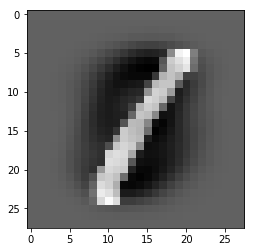In :
p = X[3, :]@ V[:, :10]

In :
recon = V[:, :10] @ p + mean

In :
plt.imshow(recon.reshape((28,28)), cmap='gray')

Out:
<matplotlib.image.AxesImage at 0x7f5f82961f28>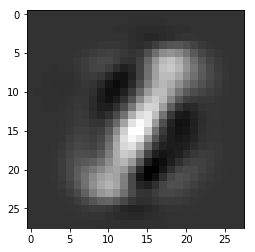In :
plt.figure(figsize=(10,10))
for i in range(100):
plt.subplot(10, 10, i+1)
plt.imshow(V[:,i].reshape((28,28)), cmap='gray')
plt.axis('off')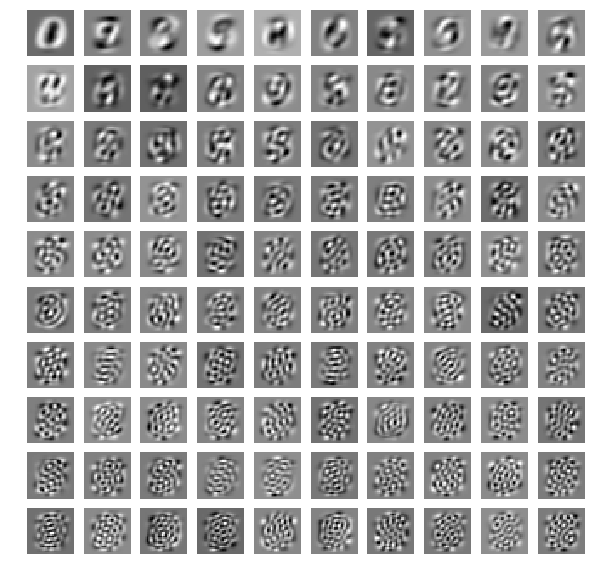In :
V.shape

Out:
(784, 784)
In :
plt.figure(figsize=(10,10))
for i in range(100):
plt.subplot(10, 10, i+1)
plt.imshow(V[:, 680+i].reshape((28,28)), cmap='gray')
plt.axis('off')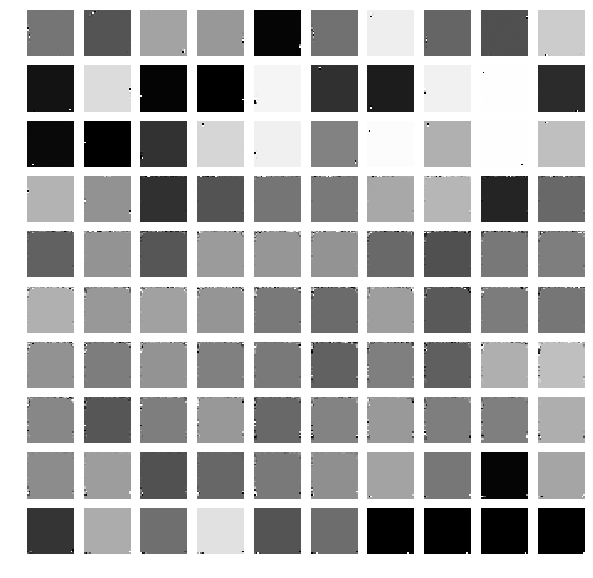In :
import sys
sys.path = ['.'] + sys.path

import neuralnetworks as nn

nnet = nn.NeuralNetworkClassifier(784, , 10)
nnet.train(Xtrain, Ttrain, 100, verbose=True)

SCG: Iteration 10 fValue 0.029047325306025797 Scale 1.953125e-09
SCG: Iteration 20 fValue 0.019683865048739275 Scale 1.9073486328125e-12
SCG: Iteration 30 fValue 0.009901197072319982 Scale 1.862645149230957e-15
SCG: Iteration 40 fValue 0.002780034273408134 Scale 1e-15
SCG: Iteration 50 fValue 0.00040800722243969313 Scale 1e-15
SCG: Iteration 60 fValue 7.239667240461288e-05 Scale 1e-15
SCG: Iteration 70 fValue 6.318047560007929e-05 Scale 6.5536e-11
SCG: Iteration 80 fValue 5.879011549287683e-05 Scale 6.8719476736e-05
SCG: Iteration 90 fValue 4.366649442508245e-05 Scale 0.000274877906944
SCG: Iteration 100 fValue 4.206540032433731e-05 Scale 0.002199023255552

Out:
NeuralNetwork(784, , 10)
Network was trained for 100 iterations. Final error is 4.206540032433731e-05.
In :
plt.plot(nnet.getErrorTrace());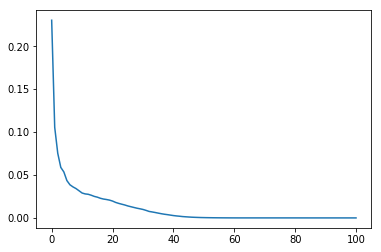In :
def percentCorrect(a, b):
return np.sum(a == b) / len(a) * 100

In :
print('% Correct: Train {:.2f} Test {:.2f}'.format(percentCorrect(Ttrain, nnet.use(Xtrain)),
percentCorrect(Ttest, nnet.use(Xtest))))

% Correct: Train 100.00 Test 96.44

In :
Xtrain = Xtrain - mean
Xtest = Xtest - mean

In :
nnet = nn.NeuralNetworkClassifier(3, , 10)
nnet.train(Xtrain @ V[:, :3], Ttrain, 100, verbose=True)

SCG: Iteration 10 fValue 0.13279651555575142 Scale 1.953125e-09
SCG: Iteration 20 fValue 0.12719967604977878 Scale 1.9073486328125e-12
SCG: Iteration 30 fValue 0.12437094907454489 Scale 1.862645149230957e-15
SCG: Iteration 40 fValue 0.1229509370649533 Scale 1e-15
SCG: Iteration 50 fValue 0.12164514805504575 Scale 1e-15
SCG: Iteration 60 fValue 0.12081401125448739 Scale 1e-15
SCG: Iteration 70 fValue 0.12017249332774405 Scale 1e-15
SCG: Iteration 80 fValue 0.11964620014593964 Scale 1e-15
SCG: Iteration 90 fValue 0.11924124932524847 Scale 1e-15
SCG: Iteration 100 fValue 0.11885113006643229 Scale 1e-15

Out:
NeuralNetwork(3, , 10)
Network was trained for 100 iterations. Final error is 0.11885113006643229.
In :
print('% Correct: Train {:.2f} Test {:.2f}'.format(percentCorrect(Ttrain, nnet.use(Xtrain @ V[:, :3])),
percentCorrect(Ttest, nnet.use(Xtest @ V[:, :3]))))

% Correct: Train 51.94 Test 53.21

In :
results = []
for nComponents in range(1, 60, 2):
nnet = nn.NeuralNetworkClassifier(nComponents, , 10)
nnet.train(Xtrain @ V[:, :nComponents], Ttrain, 200)
Ytrain = nnet.use(Xtrain @ V[:, :nComponents])
Ytest = nnet.use(Xtest @ V[:, :nComponents])
result = [nComponents, percentCorrect(Ttrain, Ytrain), percentCorrect(Ttest, Ytest)]
results.append(result)
print('nComponents {} %Correct Train {:.2f} Test {:.2f}'.format(nComponents,
percentCorrect(Ttrain, Ytrain),
percentCorrect(Ttest, Ytest)))

nComponents 1 %Correct Train 30.49 Test 31.51
nComponents 3 %Correct Train 52.32 Test 53.28
nComponents 5 %Correct Train 76.49 Test 77.09
nComponents 7 %Correct Train 89.39 Test 88.68
nComponents 9 %Correct Train 93.59 Test 92.39
nComponents 11 %Correct Train 95.89 Test 93.95
nComponents 13 %Correct Train 97.38 Test 95.20
nComponents 15 %Correct Train 98.32 Test 95.49
nComponents 17 %Correct Train 98.82 Test 95.38
nComponents 19 %Correct Train 99.14 Test 95.90
nComponents 21 %Correct Train 99.31 Test 95.65
nComponents 23 %Correct Train 99.36 Test 95.67
nComponents 25 %Correct Train 99.51 Test 95.79
nComponents 27 %Correct Train 99.56 Test 95.94
nComponents 29 %Correct Train 99.57 Test 96.17
nComponents 31 %Correct Train 99.72 Test 95.85
nComponents 33 %Correct Train 99.87 Test 96.07
nComponents 35 %Correct Train 99.76 Test 95.89
nComponents 37 %Correct Train 99.92 Test 95.92
nComponents 39 %Correct Train 99.78 Test 96.07
nComponents 41 %Correct Train 99.98 Test 96.18
nComponents 43 %Correct Train 99.66 Test 95.73
nComponents 45 %Correct Train 99.79 Test 95.84
nComponents 47 %Correct Train 99.79 Test 95.83
nComponents 49 %Correct Train 99.92 Test 96.23
nComponents 51 %Correct Train 99.72 Test 95.68
nComponents 53 %Correct Train 99.97 Test 96.30
nComponents 55 %Correct Train 99.66 Test 95.68
nComponents 57 %Correct Train 99.95 Test 96.08
nComponents 59 %Correct Train 99.98 Test 95.78

In :
results = np.array(results)
plt.plot(results[:, 0], results[:, 1], label='Train % Correct')
plt.plot(results[:, 0], results[:, 2], label='Test % Correct')
plt.legend()
plt.xlabel('Number of PCA Components Used');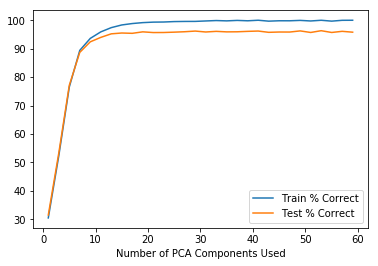## Compare to regular neural net with 20 units in first layer.¶

In :
nnet = nn.NeuralNetworkClassifier(784, [20, 100], 10)
nnet.train(Xtrain, Ttrain, 500, verbose=True)
nnet

SCG: Iteration 50 fValue 0.015063229399580188 Scale 1e-15
SCG: Iteration 100 fValue 0.003971020853287487 Scale 1e-15
SCG: Iteration 150 fValue 0.0011941483060338826 Scale 1.0600133272604741e-08
SCG: Iteration 200 fValue 0.0003335106675940921 Scale 1e-15
SCG: Iteration 250 fValue 8.166327695633968e-05 Scale 1e-15
SCG: Iteration 300 fValue 7.091768098874483e-05 Scale 4611.686018427388
SCG: Iteration 350 fValue 7.029139309283508e-05 Scale 2.251799813685248
SCG: Iteration 400 fValue 6.308472455393662e-05 Scale 0.008796093022208
SCG: Iteration 450 fValue 6.244964588445394e-05 Scale 151115727.45182866
SCG: Iteration 500 fValue 6.244964588445039e-05 Scale 1e+20

Out:
NeuralNetwork(784, [20, 100], 10)
Network was trained for 500 iterations. Final error is 6.244964588445039e-05.
In :
plt.plot(nnet.getErrorTrace());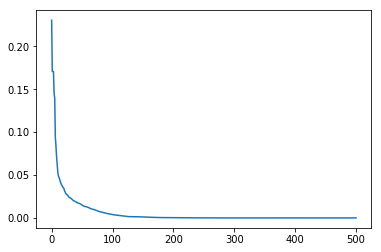In :
Ytrain = nnet.use(Xtrain)
Ytest = nnet.use(Xtest)

print('%correct train {:.2f} test {:.2f}'.format(
percentCorrect(Ytrain,Ttrain), percentCorrect(Ytest, Ttest)))

%correct train 100.00 test 92.86


The weights in the first layer, of 20 units, can be reshaped into images to see what patterns they detect in the digits.

In :
plt.figure(figsize=(10,10))
for i in range(20):
plt.subplot(5, 5, i+1)
plt.imshow(nnet.Vs[1:, i].reshape((28,28)), cmap='gray')
plt.axis('off')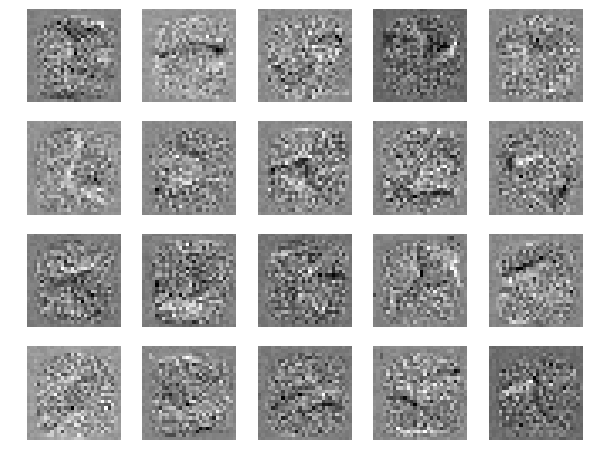Here are the first 20 singular vectors again.

In :
plt.figure(figsize=(10,10))
for i in range(20):
plt.subplot(5, 5, i+1)
plt.imshow(V[:,i].reshape((28,28)), cmap='gray')
plt.axis('off')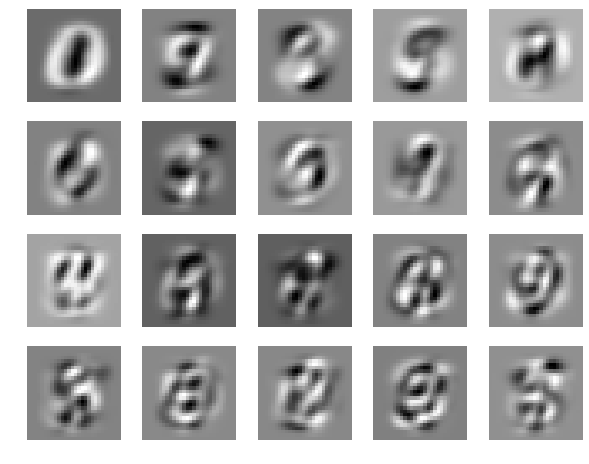In [ ]: